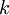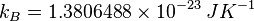2022: SklogWiki celebrates 15 years on-line

# Boltzmann constant

The Boltzmann constant ($k$ or$k_B$) is the physical constant relating temperature to energy. It is named after the Austrian physicist Ludwig Eduard Boltzmann. Its experimentally determined value (in SI units, 2010 CODATA value) is:$k_B =1.3806488 \times 10^{-23} \left. JK^{-1}\right.$

In units with molecular significance it is close to 1, for example see: DL_POLY units.

## History of Boltzmann's constant

"This constant is often referred to as Boltzmann's constant, although, to my knowledge, Boltzmann himself never introduced it - a peculiar state of affairs, which can be explained by the fact that Boltzmann, as appears from his occasional utterances, never gave thought to the possibility of carrying out an exact measurement of the constant."

Max Planck, Nobel Lecture, June 2, 1920

## Experimental determination of Boltzmann's constant

Boltzmann's constant can be obtained from the ratio of the molar gas constant to the Avogadro constant  . The molar gas constant can be obtained via acoustic gas thermometry, and Avogadros constant from either the Silicon sphere, or via the watt balance. Recently laser spectroscopy has been used to determine the constant  . Other techniques include Coulomb blockade thermometry  .